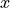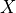# Continuous¶

## Canonical Factor¶

The intermediate factors in a Gaussian network can be described compactly using a simple parametric representation called the canonical form. This representation is closed under the basic operations used in inference: factor product, factor division, factor reduction, and marginalization. Thus, we define this CanonicalDistribution class that allows the inference process to be performed on joint Gaussian networks.

A canonical form C (X; K,h,g) is defined as

C (X; K,h,g) = exp( ((-1/2) * X.T * K * X) + (h.T * X) + g)

References

Probabilistic Graphical Models, Principles and Techniques, Daphne Koller and Nir Friedman, Section 14.2, Chapter 14.

## Continuous Factor¶

class pgmpy.factors.continuous.ContinuousFactor.ContinuousFactor(variables, pdf, *args, **kwargs)[source]

Base class for factors representing various multivariate representations.

assignment(*args)[source]

Returns a list of pdf assignments for the corresponding values.

Parameters:

*args (values) – Values whose assignment is to be computed.

Examples

>>> from pgmpy.factors.continuous import ContinuousFactor
>>> from scipy.stats import multivariate_normal
>>> normal_pdf = lambda x1, x2: multivariate_normal.pdf((x1, x2), [0, 0], [[1, 0], [0, 1]])
>>> phi = ContinuousFactor(['x1', 'x2'], normal_pdf)
>>> phi.assignment(1, 2)
0.013064233284684921

copy()[source]

Return a copy of the distribution.

Returns:

ContinuousFactor object

Return type:

copy of the distribution

Examples

>>> import numpy as np
>>> from scipy.special import beta
>>> from pgmpy.factors.continuous import ContinuousFactor
# Two variable dirichlet distribution with alpha = (1,2)
>>> def dirichlet_pdf(x, y):
...     return (np.power(x, 1) * np.power(y, 2)) / beta(x, y)
>>> dirichlet_factor = ContinuousFactor(['x', 'y'], dirichlet_pdf)
>>> dirichlet_factor.variables
['x', 'y']
>>> copy_factor = dirichlet_factor.copy()
>>> copy_factor.variables
['x', 'y']

discretize(method, *args, **kwargs)[source]

Discretizes the continuous distribution into discrete probability masses using various methods.

Parameters:
• method (A Discretizer Class from pgmpy.discretize) –

• *args – The parameters to be given to the Discretizer Class.

• **kwargs – The parameters to be given to the Discretizer Class.

Returns:

• An n-D array or a DiscreteFactor object according to the discretiztion

• method used.

Examples

>>> import numpy as np
>>> from scipy.special import beta
>>> from pgmpy.factors.continuous import ContinuousFactor
>>> from pgmpy.factors.continuous import RoundingDiscretizer
>>> def dirichlet_pdf(x, y):
...     return (np.power(x, 1) * np.power(y, 2)) / beta(x, y)
>>> dirichlet_factor = ContinuousFactor(['x', 'y'], dirichlet_pdf)
>>> dirichlet_factor.discretize(RoundingDiscretizer, low=1, high=2, cardinality=5)
# TODO: finish this

divide(other, inplace=True)[source]

Gives the ContinuousFactor divide with the other factor.

Parameters:

other (ContinuousFactor) – The ContinuousFactor to be divided.

Returns:

if inplace=True (default) returns None if inplace=False returns a new ContinuousFactor instance.

Return type:

Examples

>>> from pgmpy.factors.continuous import ContinuousFactor
>>> from scipy.stats import multivariate_normal
>>> sn_pdf1 = lambda x: multivariate_normal.pdf([x], , [])
>>> sn_pdf2 = lambda x1,x2: multivariate_normal.pdf([x1, x2], [0, 0], [[1, 0], [0, 1]])
>>> sn1 = ContinuousFactor(['x2'], sn_pdf1)
>>> sn2 = ContinuousFactor(['x1', 'x2'], sn_pdf2)

>>> sn4 = sn2.divide(sn1, inplace=False)
>>> sn4.assignment(0, 0)
0.3989422804014327

>>> sn4 = sn2 / sn1
>>> sn4.assignment(0, 0)
0.3989422804014327

marginalize(variables, inplace=True)[source]

Marginalize the factor with respect to the given variables.

Parameters:
• variables (list, array-like) – List of variables with respect to which factor is to be maximized.

• inplace (boolean) – If inplace=True it will modify the factor itself, else would return a new ContinuousFactor instance.

Returns:

DiscreteFactor or None – if inplace=False returns a new ContinuousFactor instance.

Return type:

if inplace=True (default) returns None

Examples

>>> from pgmpy.factors.continuous import ContinuousFactor
>>> from scipy.stats import multivariate_normal
>>> std_normal_pdf = lambda *x: multivariate_normal.pdf(x, [0, 0], [[1, 0], [0, 1]])
>>> std_normal = ContinuousFactor(['x1', 'x2'], std_normal_pdf)
>>> std_normal.scope()
['x1', 'x2']
>>> std_normal.assignment([1, 1])
0.058549831524319168
>>> std_normal.marginalize(['x2'])
>>> std_normal.scope()
['x1']
>>> std_normal.assignment(1)

normalize(inplace=True)[source]

Normalizes the pdf of the continuous factor so that it integrates to 1 over all the variables.

Parameters:

inplace (boolean) – If inplace=True it will modify the factor itself, else would return a new factor.

Returns:

if inplace=True (default) returns None if inplace=False returns a new ContinuousFactor instance.

Return type:

Examples

>>> from pgmpy.factors.continuous import ContinuousFactor
>>> from scipy.stats import multivariate_normal
>>> std_normal_pdf = lambda x: 2 * multivariate_normal.pdf(x, [0, 0], [[1, 0], [0, 1]])
>>> std_normal = ContinuousFactor(['x1', 'x2'], std_normal_pdf)
>>> std_normal.assignment(1, 1)
0.117099663049
>>> std_normal.normalize()
>>> std_normal.assignment(1, 1)
0.0585498315243

property pdf

Returns the pdf of the ContinuousFactor.

product(other, inplace=True)[source]

Gives the ContinuousFactor product with the other factor.

Parameters:

other (ContinuousFactor) – The ContinuousFactor to be multiplied.

Returns:

if inplace=True (default) returns None if inplace=False returns a new ContinuousFactor instance.

Return type:

Examples

>>> from pgmpy.factors.continuous import ContinuousFactor
>>> from scipy.stats import multivariate_normal
>>> sn_pdf1 = lambda x: multivariate_normal.pdf([x], , [])
>>> sn_pdf2 = lambda x1,x2: multivariate_normal.pdf([x1, x2], [0, 0], [[1, 0], [0, 1]])
>>> sn1 = ContinuousFactor(['x2'], sn_pdf1)
>>> sn2 = ContinuousFactor(['x1', 'x2'], sn_pdf2)

>>> sn3 = sn1.product(sn2, inplace=False)
>>> sn3.assignment(0, 0)
0.063493635934240983

>>> sn3 = sn1 * sn2
>>> sn3.assignment(0, 0)
0.063493635934240983

reduce(values, inplace=True)[source]

Reduces the factor to the context of the given variable values.

Parameters:
• values (list, array-like) – A list of tuples of the form (variable_name, variable_value).

• inplace (boolean) – If inplace=True it will modify the factor itself, else would return a new ContinuousFactor object.

Returns:

ContinuousFactor or None – if inplace=False returns a new ContinuousFactor instance.

Return type:

if inplace=True (default) returns None

Examples

>>> import numpy as np
>>> from scipy.special import beta
>>> from pgmpy.factors.continuous import ContinuousFactor
>>> def custom_pdf(x, y, z):
...     return z*(np.power(x, 1) * np.power(y, 2)) / beta(x, y)
>>> custom_factor = ContinuousFactor(['x', 'y', 'z'], custom_pdf)
>>> custom_factor.variables
['x', 'y', 'z']
>>> custom_factor.assignment(1, 2, 3)
24.0

>>> custom_factor.reduce([('y', 2)])
>>> custom_factor.variables
['x', 'z']
>>> custom_factor.assignment(1, 3)
24.0

scope()[source]

Returns the scope of the factor.

Returns:

list

Return type:

List of variable names in the scope of the factor.

Examples

>>> from pgmpy.factors.continuous import ContinuousFactor
>>> from scipy.stats import multivariate_normal
>>> normal_pdf = lambda x: multivariate_normal(x, [0, 0], [[1, 0], [0, 1]])
>>> phi = ContinuousFactor(['x1', 'x2'], normal_pdf)
>>> phi.scope()
['x1', 'x2']


## Linear Gaussian CPD¶

class pgmpy.factors.continuous.LinearGaussianCPD.LinearGaussianCPD(variable, evidence_mean, evidence_variance, evidence=[], beta=None)[source]

For, X -> Y the Linear Gaussian model assumes that the mean of Y is a linear function of mean of X and the variance of Y does not depend on X.

For example,Here,is the mean of the variable.

Letbe a continuous variable with continuous parents. We say thathas a linear Gaussian CPD if there are parametersandsuch that,

In vector notation,

References

copy()[source]

Returns a copy of the distribution.

Returns:

LinearGaussianCPD

Return type:

copy of the distribution

Examples

>>> from pgmpy.factors.continuous import LinearGaussianCPD
>>> cpd = LinearGaussianCPD('Y',  [0.2, -2, 3, 7], 9.6, ['X1', 'X2', 'X3'])
>>> copy_cpd = cpd.copy()
>>> copy_cpd.variable
'Y'
>>> copy_cpd.evidence
['X1', 'X2', 'X3']

fit(data, states, estimator=None, complete_samples_only=True, **kwargs)[source]

Determine βs from data

Parameters:
• data (pandas.DataFrame) – Dataframe containing samples from the conditional distribution, p(Y|X) estimator: ‘MLE’ or ‘MAP’

• completely_samples_only (boolean (True or False)) – Are they downsampled or complete? Defaults to True

maximum_likelihood_estimator(data, states)[source]

Fit using MLE method.

Parameters:
• data (pandas.DataFrame or 2D array) – Dataframe of values containing samples from the conditional distribution, (Y|X) and corresponding X values.

• states (All the input states that are jointly gaussian.) –

Returns:

beta, variance (tuple)

Return type:

Returns estimated betas and the variance.